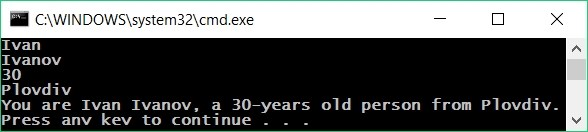# Printing and Formatting Text and Numbers

In C#, when printing a text, numbers and other data on the console, we can join them by using templates {0}, {1}, {2} etc. In programming, these templates are called placeholders. This is a simple example:

Console.WriteLine("{0} + {1} = {2}", 3, 5, 3+5);


The placeholders {0}, {1} and {2} are replaced by the expressions, given after the text. The result from the above code is:

3 + 5 = 8


## Video: Printing Text and Numbers

Watch a video lesson about how to print text and numbers together on the console: https://youtu.be/tSTwwaQpy9g.

## Example: Printing Text and Numbers

var firstName = Console.ReadLine();
Console.WriteLine("You are {0} {1}, a {2}-years old person from {3}.",
firstName, lastName, age, town);


This is the result we are going to obtain after the execution of this example:Notice how every variable should be passed in the order, in which we want it to be printed. Practically, the template (placeholder) accepts variables of any type.

It is possible for a template to be used multiple times and it is not necessary for the templates to be numbered sequentially. Here is an example:

Console.WriteLine("{1} + {1} = {0}", 1+1, 1);


The result is:

1 + 1 = 2


## Using the Dollar String Interpolation

We can format text in C# using also the following $syntax. It provides simplifies text formatting: var a = 4.5; Console.WriteLine($"Square size = {a}");
Console.WriteLine($"Square area = {a * a}");  The output from the above code is as follows: Square size = 4.5 Square area = 20.25  The $ prefix before a string in C# enables the so called "string interpolation": replacing all expressions, staying in curly brackets { } in the text with their values.

Using the dollar string interpolation syntax, the last example can be rewritten like this:

var firstName = Console.ReadLine();